# Real Estate Principles Study Set 1

## Quiz 8 : Valuation Using the Income ApproachStudy FlashcardsLooking for Insurance Homework Help?

## Quiz 8 :Valuation Using the Income Approach

Question TypeAn overall capitalization rate ( R o ) is divided into which type of income or cash flow to obtain an indicated market value
Free
Multiple Choice

The market valuation approach of finding market value of property through income approach includes discounted cash flow analysis, direct capitalization, and effective gross income multiples.
The correct answer is option a.
Explanation
Effective gross income is used to find the market value of property in effective gross income multiples method, and here, discounting of income is not required to find the market value of the property.
Thus, the option b is incorrect.
The discounting of cash flows using an appropriate discount rate estimated by analysts to find the market value of asset is known as discounted cash flow analysis. Here, the discounting rate might be expected yield or required rate of return from the property.
Thus, the option c. and option d. are incorrect.
PGI (Potential Gross Income) is used to find the effective gross income and it is not used to determine market value of property directly. The effective gross income is the difference between the PGI and vacancy and collection losses.
Thus, the option e. is incorrect.
In order to find the market value of property as per the direct capitalization method the projected net operating income over the next 12 months will be divided by the overall capitalization rate.
Thus, the correct answer is option a.

Tags
Choose question tagThe final value estimate produced by one approach is called:
Free
Multiple Choice

Option "a" final estimate of value represents the actual worth of any asset after depreciation and other cost associated with that particular asset. Thus this option is not correct.
Option "b" reconciled estimate of value represents the modified and corrected worth or value of any assets after rectifying the principle errors in the valuation of that asset. Thus this option is not correct.
Option "c" probable sale price refers to the expected price to be set as the selling price of any asset or tangible product. Thus probable sale price is the price which is surely be received by the seller for selling the property, land or some other asset to buyer. Thus this option is not correct.
Option "e" adjusted final sale price refers to the final price set as the selling price for any fixed asset by the seller after being negotiated with the buyer of that particular asset on the features and characteristics of that asset. Thus this option is not correct.
Option "d" indicated value refers to the value shown as a final estimate by marketing approaches to determine the price and worth of a particular asset or other fixed property. Thus this option is correct.
Hence the correct option is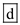that is indicated value

Tags
Choose question tagYou are estimating the market value of a small office building. Suppose the estimated NOI for the first year of operations is $100,000. a. If you expect that NOI will remain constant at$100,000 over the next 50 years and that the office building will have no value at the end of 50 years, what is the present value of the building assuming a 12.2% discount rate If you pay this amount, what is the indicated initial cap rate b. If you expect that NOI will remain constant at $100,000 forever, what is the value of the building assuming a 12.2% discount rate If you pay this amount, what is the indicated initial cap rate c. If you expect that the initial$100,000 NOI will grow forever at a 3% annual rate, what is the value of the building assuming a 12.2% discount rate If you pay this amount, what is the indicated initial cap rate
Free
Essay

We are asked to use the following information to determine a solution:
You are estimating the value of a small office building. Suppose the estimated NOI for the first year of operations is $100,000. (a) Calculate the present value of the building and indicated initial cap rate: If you expect that NOI will remain constant at$100,000 over the next 50 years and that the office building will have no value at the end of 50 years, what is the present value of the building assuming a 12.2% discount rate If you pay this amount, what is the indicated initial cap rate
In order to determine the present value , using your financial calculator and plugin the values as shown in below table:
Table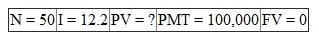Hence the present value is $817,078. In order to determine the initial (going-in) cap rate we need to divide the payment amount by the present value.Hence the initial cap rate is 12.24%. (b) Calculate the value of the building and indicated initial cap rate: If you expect that NOI will remain constant at$100,000 forever, what is the value of the building assuming a 12.2% discount rate If you pay this amount, what is the indicated initial cap rate
The value of the building with NOI remaining constant at $100,000 is calculated using the formula for perpetuity.Hence the value of the building is$819,672.00.
In order to determine the indicated initial cap rate we need to divide the payment amount by the value of the building.Hence the initial cap rate is 12.2%.
(c) Calculate the value of the building and indicated initial cap rate:
If you expect the initial $100,000 NOI will grow forever at a 3% annual rate, what is the value of the building assuming a 12.2% discount rate If you pay this amount, what is the indicated initial cap rate In order to determine the value of the building we need to determine the initial cap rate by subtracting the annual rate from the discount rate.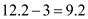Hence the initial cap rate is 9.2%. In order to determine the value of the building we need to divide the payment by the initial cap rate.Hence the indicated value of the building is$1,086,957.

Tags
Choose question tagThe methodology of appraisal differs from that of investment analysis primarily regarding:
Multiple Choice
Tags
Choose question tagUse the following information to answer questions 9 and 10. You have just completed the appraisal of an office building and have concluded that the market value of the property is $2,500,000. You expect potential gross income (PGI) in the first year of operations to be$450,000; vacancy and collection losses to be 9 percent of PGI; operating expenses to be 38 percent of effective gross income (EGI); and capital expenditures to be 4 percent of EGI. What is the implied going-in capitalization rate
Multiple Choice
Tags
Choose question tagData for five comparable income properties that sold recently are shown in the accompanying table.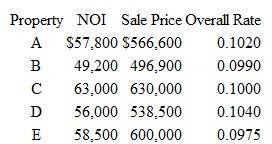What is the indicated overall cap rate ( Ro )
Essay
Tags
Choose question tagUse the following information to answer questions 9 and 10. You have just completed the appraisal of an office building and have concluded that the market value of the property is $2,500,000. You expect potential gross income (PGI) in the first year of operations to be$450,000; vacancy and collection losses to be 9 percent of PGI; operating expenses to be 38 percent of effective gross income (EGI); and capital expenditures to be 4 percent of EGI. What is the effective gross income multiplier (EGIM)
Multiple Choice
Tags
Choose question tagDistinguish between levered and unlevered cash flows. In what sense does the equity investor have a residual claim on the property's cash flow stream if mortgage financing is employed
Essay
Tags
Choose question tagWhy is the market value of real estate determined partly by the lender's requirements and partly by the requirements of equity investors
Essay
Tags
Choose question tagAssume a reserve for nonrecurring capital expenditures is to be included in the pro forma for the subject property. Explain how an above-line treatment of this expenditure would differ from a below-line treatment.
Essay
Tags
Choose question tagWhich of the following types of properties probably would not be appropriate for income capitalization
Multiple ChoiceYou have been asked to estimate the market value of an apartment complex that is producing annual net operating income of $44,500. Four highly similar and competitive apartment properties within two blocks of the subject property have sold in the past three months. All four offer essentially the same amenities and services as the subject. All were open-market transactions with similar terms of sale. All were financed with 30-year fixed-rate mortgages using 70 percent debt and 30 percent equity. The sale prices and estimated first year net operating incomes were as follows: Comparable 1: Sales price$500,000; NOI $55,000 Comparable 2: Sales price$420,000; NOI $50,400 Comparable 3: Sales price$475,000; NOI $53,400 Comparable 4: Sales price$600,000; NOI $69,000 What is the indicated value of the subject property using direct capitalization Essay Answer: Tags Choose question tagGiven the following owner's income and expense estimates for an apartment property, formulate a reconstructed operating statement. The building consists of 10 units that could rent for$550 per month each.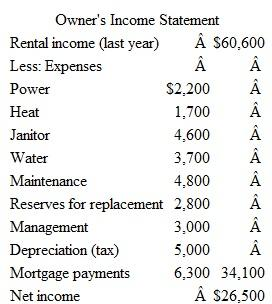Estimating vacancy and collection losses at 5 percent of potential gross income, reconstruct the operating statement to obtain an estimate of NOI. Remember, there may be items in the owner's statement that should not be included in the reconstructed operating statement. Using the NOI and an R 0 of 11.0 percent, calculate the property's indicated market value. Round your final answer to the nearest $500. Essay Answer: Tags Choose question tagDescribe the conditions under which the use of gross income multipliers to value the subject property is appropriate. Essay Answer: Tags Choose question tagUse the following property data: Cash flow from operations: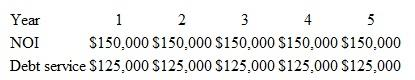Cash flow at sale: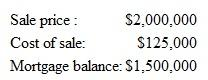a. Assuming the going-in capitalization rate is 8 percent, compute a value for the property using direct capitalization. b. Assuming the required return on unlevered cash flows is 10 percent, and that the property will be held by a buyer for five years, compute the value of the property based on discounting unlevered cash flows. c. Assuming the relevant required return on levered cash flows is 15 percent, and that the property will be held by a buyer for five years, what is the present value of the levered cash flows Essay Answer: Tags Choose question tagIn what situations or for which types of properties might discounted cash flow analysis be preferred to direct capitalization Essay Answer: Tags Choose question tagReserves for replacement and other nonrecurring expenses are allowances that reflect: Multiple Choice Answer: Tags Choose question tagWhich of the following expenses is not an operating expense Multiple Choice Answer: Tags Choose question tagIf a comparable property sells for$1,200,000 and the effective gross income of the property is $12,000 per month, the effective gross income multiplier is: Multiple Choice Answer: Tags Choose question tagAn appraiser estimates that a property will produce NOI of$25,000, the y 0 is 11 percent, and the growth rate is 2.0 percent. What is the total property value (unrounded)